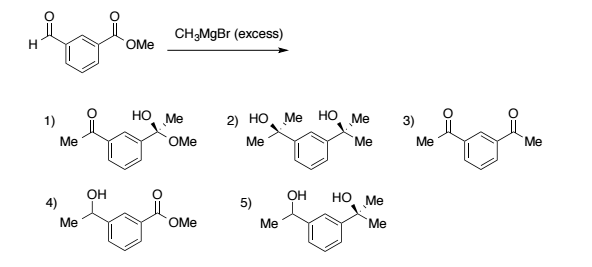# Problem: Predict the product of the following reaction. Assume an acid quench.

###### FREE Expert Solution
82% (219 ratings)
###### Problem Details

Predict the product of the following reaction. Assume an acid quench.Frequently Asked Questions

What scientific concept do you need to know in order to solve this problem?

Our tutors have indicated that to solve this problem you will need to apply the Grignard Reaction concept. You can view video lessons to learn Grignard Reaction. Or if you need more Grignard Reaction practice, you can also practice Grignard Reaction practice problems.

What is the difficulty of this problem?

Our tutors rated the difficulty ofPredict the product of the following reaction. Assume an aci...as high difficulty.

How long does this problem take to solve?

Our expert Organic tutor, Jonathan took 2 minutes and 49 seconds to solve this problem. You can follow their steps in the video explanation above.